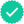#### Related questions#### Concept explainers

Question

Expert SolutionStep 1

Consider the given equation and replace the term cos(4x) using double angle trigonometric identity. We use, cos(4x) = 2cos2(2 x) – 1. This will help us have the entire equation in the form of cos(2x). We get:

Step 2

We now have a quadratic equation in terms of cos(2x). Hence, we can first solve for cos(2 x), by solving the equation using factoring.

Step 3

Solving further we get:Trending nowThis is a popular solution!Step by stepSolved in 6 steps with 5 imagesKnowledge BoosterRecommended textbooks for you
•Trigonometry (MindTap Course List)
Trigonometry
ISBN:9781337278461
Author:Ron Larson
Publisher:Cengage LearningAlgebra & Trigonometry with Analytic Geometry
Algebra
ISBN:9781133382119
Author:Swokowski
Publisher:Cengage
•Trigonometry (MindTap Course List)
Trigonometry
ISBN:9781337278461
Author:Ron Larson
Publisher:Cengage LearningAlgebra & Trigonometry with Analytic Geometry
Algebra
ISBN:9781133382119
Author:Swokowski
Publisher:Cengage